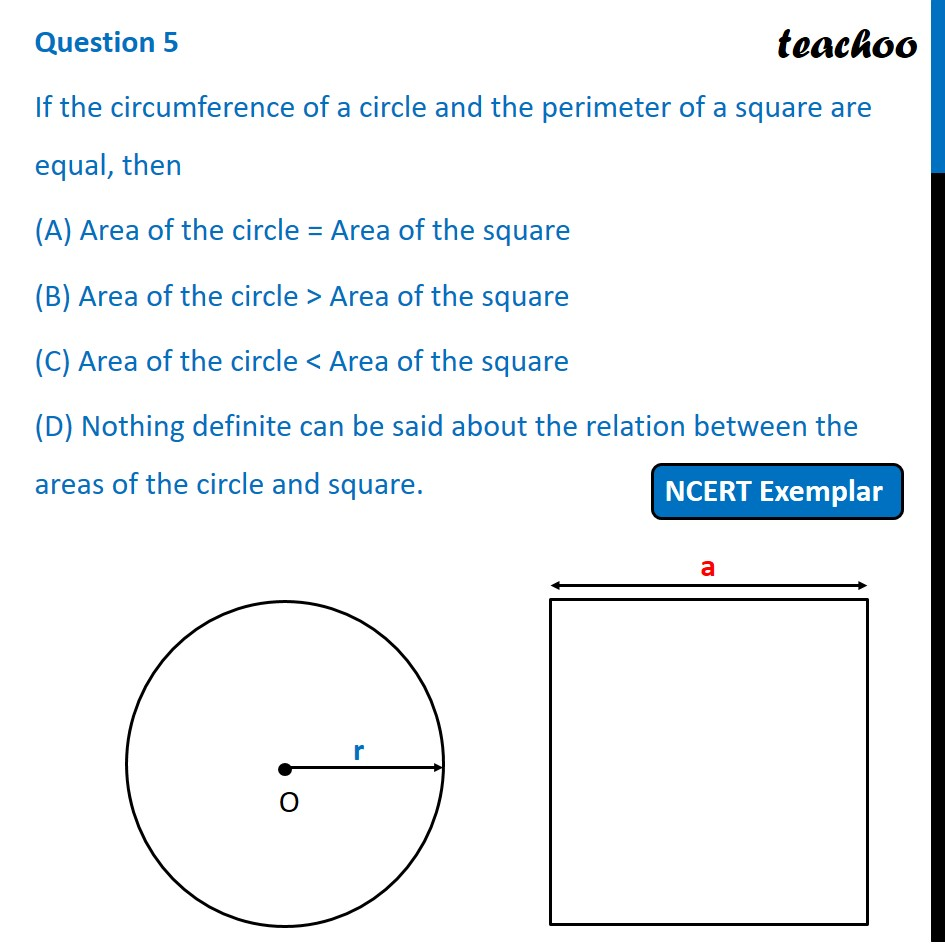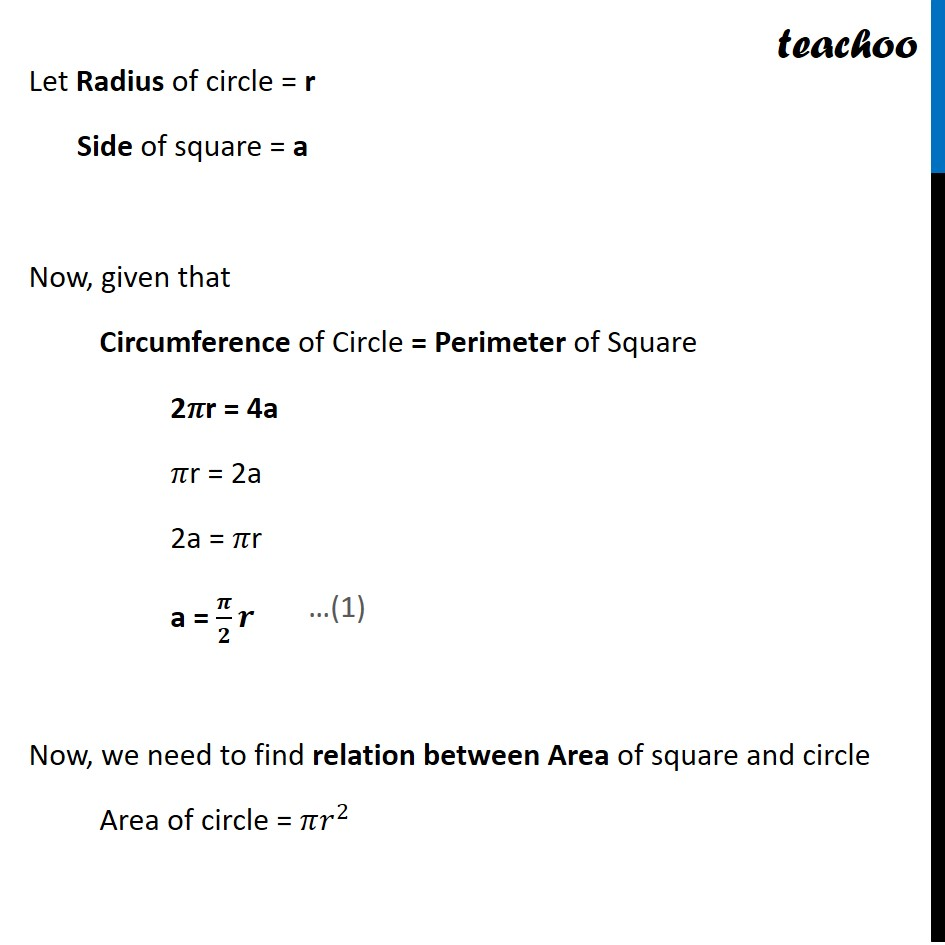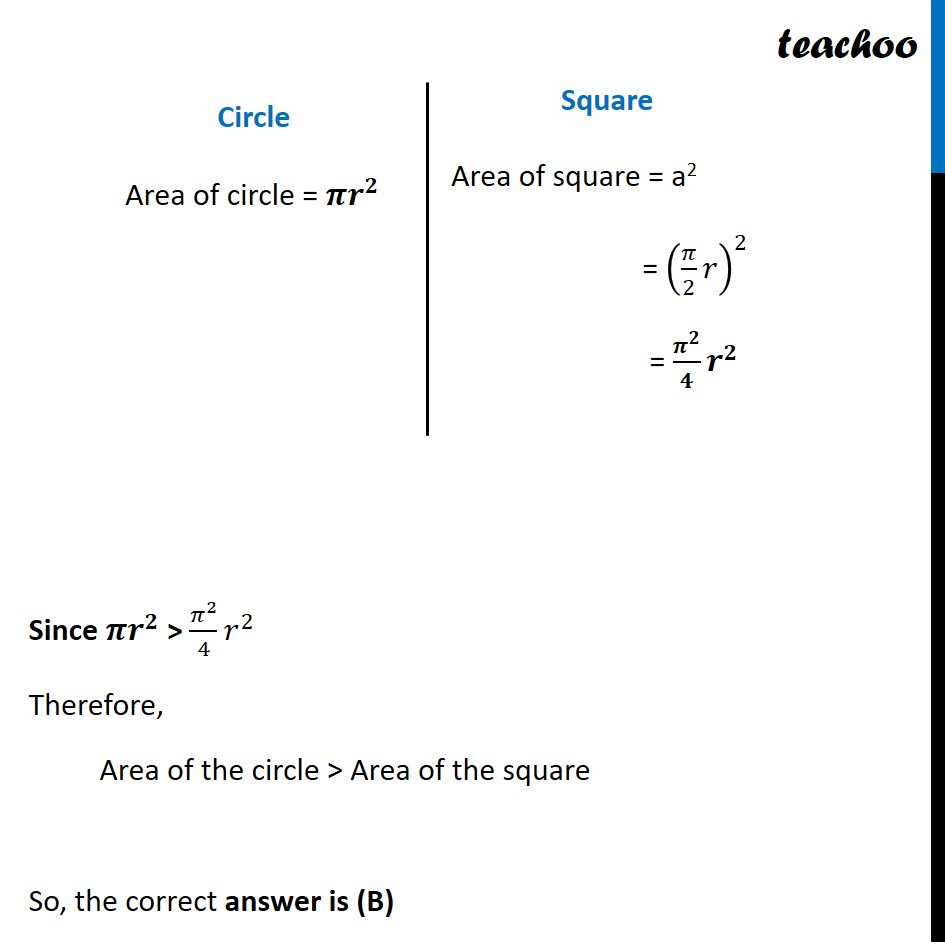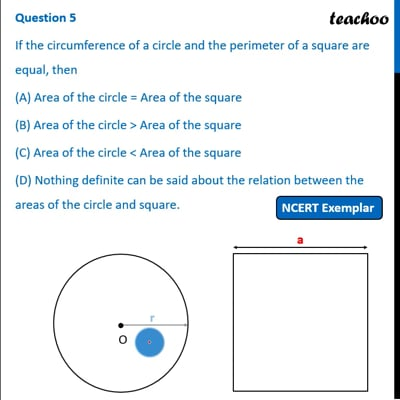NCERT Exemplar - MCQ

Chapter 11 Class 10 Areas related to Circles
Serial order wise

## (D) Nothing definite can be said about the relation between the areas of the circle and square.This video is only available for Teachoo black users

Learn in your speed, with individual attention - Teachoo Maths 1-on-1 Class

### Transcript

Question 5 If the circumference of a circle and the perimeter of a square are equal, then (A) Area of the circle = Area of the square (B) Area of the circle > Area of the square (C) Area of the circle < Area of the square (D) Nothing definite can be said about the relation between the areas of the circle and square. Let Radius of circle = r Side of square = a Now, given that Circumference of Circle = Perimeter of Square 2𝜋r = 4a 𝜋r = 2a 2a = 𝜋r a = 𝝅/𝟐 𝒓 Now, we need to find relation between Area of square and circle Area of circle = 𝜋𝑟^2 Circle Area of circle = 𝝅𝒓^𝟐 Square Area of square = a2 = (𝜋/2 𝑟)^2 = 𝝅^𝟐/𝟒 𝒓^𝟐 Since 𝝅𝒓^𝟐 > 𝜋^2/4 𝑟^2 Therefore, Area of the circle > Area of the square So, the correct answer is (B)